Calibrate XCP Characteristics

This example shows how to use the XCP protocol capability to connect and calibrate available characteristic data from a Simulink model deployed to a Windows executable. The example writes to modify the parameters of the model using TCP and direct memory access, and compares the measurements before and after calibration. XCP is a high-level protocol used for accessing and modifying internal parameters and variables of a model, algorithm, or ECU. For more information, refer to the ASAM standards.

Algorithm Overview

The algorithm used in this example is a Simulink model built and deployed as an XCP server. The model has already been compiled and is available to run in the file XCPServerSineWaveGenerator.exe. Additionally, the A2L-file XCPServerSineWaveGenerator.a2l is provided as an output of that build process. The model contains three measurements and two characteristics, accessible via XCP. Because the model is already deployed, Simulink is not required to run this example. The following image illustrates the model.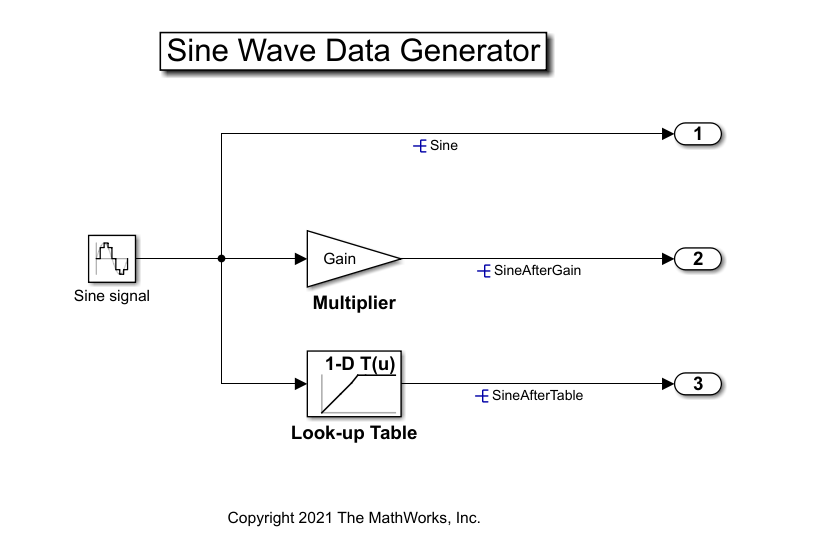The signal SineAfterGain is obtained by using the multiplier Gain to scale the source signal Sine, and the signal SineAfterTable is obtained by using the 1-D look-up table to modify the source signal Sine. Calibrating the parameter Gain and the 1-D look-up table produces different SineAfterGain and SineAfterTable waveforms, correspondingly.

For details about how to build a Simulink model, including an XCP server and generating an A2L-file, see Export ASAP2 File for Data Measurement and Calibration (Simulink Coder).

Run the XCP Server Model

To communicate with the XCP server, the deployed model must be run. By using the system function, you can execute the XCPServer.exe from inside MATLAB. The function requires constructing an argument list pointing to the executable. A separate command window opens and shows running outputs from the server.

sysCommand = ['"', fullfile(pwd, 'XCPServerSineWaveGenerator.exe'),'"', ' &'];
system(sysCommand);

Open the A2L-File

An A2L-file is required to establish a connection to the XCP server. The A2L-file describes all the functionality and capability that the XCP server provides, as well as the details of how to connect to the server. Use the xcpA2L function to open the A2L-file that describes the server model.

a2lInfo = xcpA2L("XCPServerSineWaveGenerator.a2l")
a2lInfo =
A2L with properties:

File Details
FileName: 'XCPServerSineWaveGenerator.a2l'
FilePath: 'C:\Users\siyingl\OneDrive - MathWorks\Documents\MATLAB\Examples\vnt-ex09112476\XCPServerSineWaveGenerator.a2l'
ServerName: 'ModuleName'
Warnings: [0×0 string]

Parameter Details
Events: {'100 ms'}
EventInfo: [1×1 xcp.a2l.Event]
Measurements: {'Sine'  'SineAfterGain'  'SineAfterTable'  'XCPServer_DW.lastCos'  'XCPServer_DW.lastSin'  'XCPServer_DW.systemEnable'}
MeasurementInfo: [6×1 containers.Map]
Characteristics: {'Gain'  'ydata'}
CharacteristicInfo: [2×1 containers.Map]
AxisInfo: [1×1 containers.Map]
RecordLayouts: [4×1 containers.Map]
CompuMethods: [3×1 containers.Map]
CompuTabs: [0×1 containers.Map]
CompuVTabs: [0×1 containers.Map]

XCP Protocol Details
ProtocolLayerInfo: [1×1 xcp.a2l.ProtocolLayer]
DAQInfo: [1×1 xcp.a2l.DAQ]
TransportLayerCANInfo: [0×0 xcp.a2l.XCPonCAN]
TransportLayerUDPInfo: [0×0 xcp.a2l.XCPonIP]
TransportLayerTCPInfo: [1×1 xcp.a2l.XCPonIP]

TCP is the transport protocol used to communicate with the XCP server. Details for the TCP connection, such as the IP address and port number, are contained in the TransportLayerTCPInfo property.

a2lInfo.TransportLayerTCPInfo
ans =
XCPonIP with properties:
CommonParameters: [1×1 xcp.a2l.CommonParameters]
TransportLayerInstance: ''
Port: 17725

Create an XCP Channel

To create an active XCP connection to the server, use the xcpChannel function. The function requires a reference to the server A2L-file and the type of transport protocol to use for messaging with the server.

xcpCh = xcpChannel(a2lInfo, "TCP")
xcpCh =
Channel with properties:

ServerName: 'ModuleName'
A2LFileName: 'XCPServerSineWaveGenerator.a2l'
TransportLayer: 'TCP'
TransportLayerDevice: [1×1 struct]
SeedKeyDLL: []

Connect to the Server

To make communication with the server active, use the connect function.

connect(xcpCh)

View Available Characteristics from A2L-File

A characteristic in XCP represents a tunable parameter in the memory of the model. Characteristics available for calibration are defined in the A2L-file and can be found in the Characteristics property. Note that the parameter Gain is the multiplier and ydata specifies the output data points of the 1-D look-up table.

a2lInfo.Characteristics
ans = 1×2 cell
{'Gain'}    {'ydata'}

a2lInfo.CharacteristicInfo("Gain")
ans =
Characteristic with properties:
Name: 'Gain'
LongIdentifier: ''
CharacteristicType: VALUE
Deposit: [1×1 xcp.a2l.RecordLayout]
MaxDiff: 0
Conversion: [1×1 xcp.a2l.CompuMethod]
LowerLimit: -5
UpperLimit: 5
Dimension: 1
AxisConversion: {1×0 cell}
ByteOrder: MSB_LAST
Discrete: []
Format: ''
Number: []
PhysUnit: ''

a2lInfo.CharacteristicInfo("ydata")
ans =
Characteristic with properties:
Name: 'ydata'
LongIdentifier: 'Y data'
CharacteristicType: CURVE
Deposit: [1×1 xcp.a2l.RecordLayout]
MaxDiff: 0
Conversion: [1×1 xcp.a2l.CompuMethod]
LowerLimit: -2
UpperLimit: 2
Dimension: 7
AxisConversion: {[1×1 xcp.a2l.CompuMethod]}
ByteOrder: MSB_LAST
Discrete: []
Format: ''
Number: []
PhysUnit: ''

Read the current value of the characteristic Gain. The readCharacteristic function performs a direct read from the server for a given characteristic.

initialGain = 2

Read the current 1-D look-up table characteristic using readAxis and readCharacteristic, then plot the mapping. This table effectively maps any positive input value to zero output.

inputBreakpoints = 1×7

-1.0000   -0.5000   -0.2000         0    0.2000    0.5000    1.0000

outputPoints = 1×7

-1.0000   -0.5000   -0.2000         0         0         0         0

plot(inputBreakpoints, outputPoints);
title("Initial 1-D Look-up Table Map");
xlabel("Input Value");
ylabel("Output Value");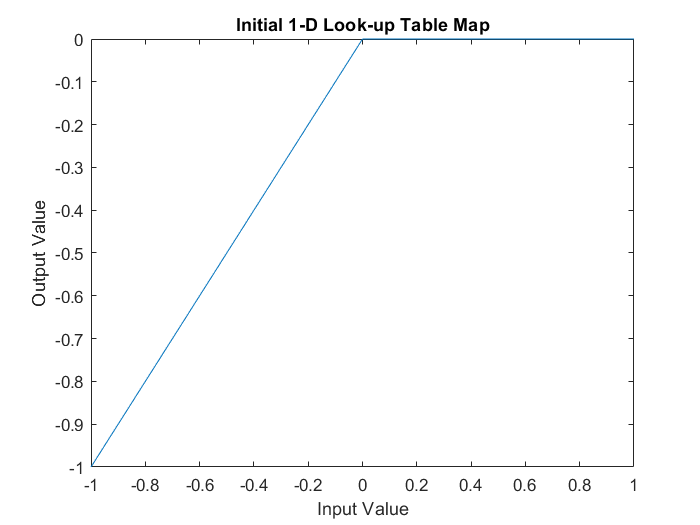Create a Measurement List

This example explores the value of the measurement Sine, unmodified and modified by the two characteristics. To visualize the continuously changing value of Sine pre- and post-calibration, acquire measurement data values using a DAQ list. Use the createMeasurementList function to create a DAQ list containing all Sine-based measurements available from the server.

createMeasurementList(xcpCh, "DAQ", "100 ms", ["Sine", "SineAfterGain", "SineAfterTable"])

Obtain Measurements Before Calibration

Use the startMeasurement function and stopMeasurement function to run the DAQ list for a short period of time.

startMeasurement(xcpCh);
pause(3);
stopMeasurement(xcpCh);

To retrieve the data acquired by the DAQ list for all the Sine-based measurements, use the readDAQ function. The number of retrieved samples during 3 seconds at 100 ms event is expected to be 30, but because the XCP server runs on Windows, which is not a real-time operating system, the actual number of retrieved samples might be less than 30, depending on how occupied the operating system is.

Inspect Measurements Before Calibration

Plot the SineAfterGain measurement against the base Sine measurement. The value after Gain is boosted by a factor of 2, based on the original measurement, because the preloaded value of the characteristic Gain is 2, as shown previously.

plot(sine, "o-"); hold on;
plot(sineAfterGain, "*-"); hold off;
title("Before Calibration: Sine Signal vs Sine Signal after Gain");
legend("Original", "After Gain");
xlabel("Data Point");
ylabel("Data Value");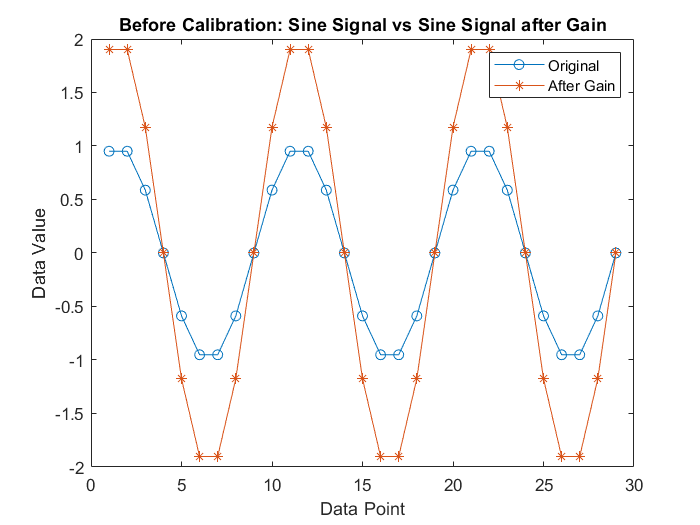Plot the SineAfterTable measurement against the base Sine measurement. Any positive value of the original measurement is mapped to zero according to the preloaded 1-D look-up table, therefore the modified signal looks truncated and does not have any positive values.

plot(sine, "o-"); hold on;
plot(sineAfterTable, "*-"); hold off;
title("Before Calibration: Sine Signal vs Sine Signal after Table");
legend("Original", "After Table");
xlabel("Data Point");
ylabel("Data Value");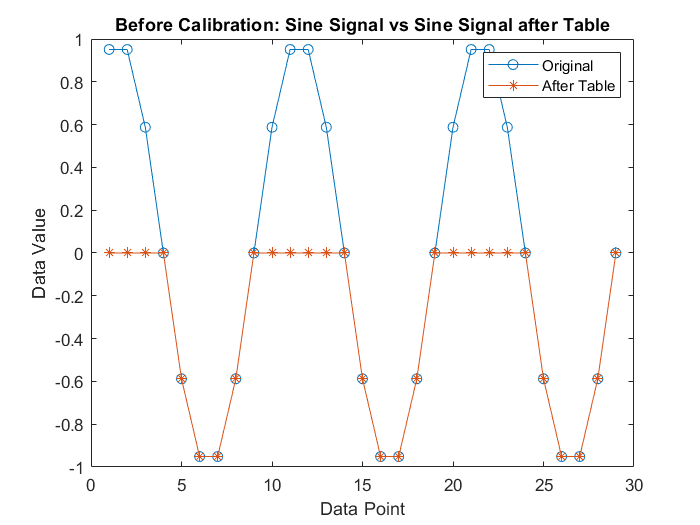Calibrate the Gain and 1-D Look-up Table

Write a new value to the charateristic Gain using writeCharacteristic, and perform a read to verify the change using readCharacteristic.

writeCharacteristic(xcpCh, "Gain", 0.5);
newGain = 0.5000

Write new data points to the output of the 1-D look-up table using writeCharacteristic.

writeCharacteristic(xcpCh, "ydata", [0 0 0 0 0.2 0.5 1]);

Read the new 1-D look-up table data using readAxis and readCharacteristic, then plot the mapping. Now the table effectively maps any negative input value to zero output.

inputBreakpoints = 1×7

-1.0000   -0.5000   -0.2000         0    0.2000    0.5000    1.0000

newOutputPoints = 1×7

0         0         0         0    0.2000    0.5000    1.0000

plot(inputBreakpoints, newOutputPoints);
title("New 1-D Look-up Table Map");
xlabel("Input Value");
ylabel("Output Value");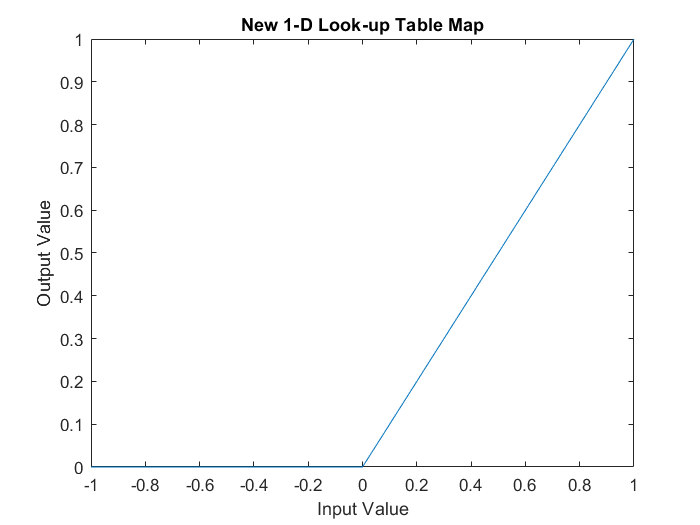Obtain Measurements after Calibration

Use the startMeasurement function and stopMeasurement function to run the DAQ list for a short period of time.

startMeasurement(xcpCh);
pause(3);
stopMeasurement(xcpCh);

To retrieve the data acquired by the DAQ list for all the Sine-based measurements, use the readDAQ function.

Inspect Measurements After Calibration

Plot the SineAfterGain measurement against the base Sine measurement. Now the value after Gain is decreased by a factor of 2, based on the original measurement, because the value of the characteristic Gain is set to 0.5 after calibration.

plot(sine, "o-"); hold on;
plot(sineAfterGain, "*-"); hold off;
title("After Calibration: Sine Signal vs Sine Signal after Gain");
legend("Original", "After Gain");
xlabel("Data Point");
ylabel("Data Value");Plot the SineAfterTable measurement against the base Sine measurement. Any negative value of the original measurement is mapped to zero according to the new 1-D look-up table, therefore the modified signal looks truncated differently and does not have any negative values.

plot(sine, "o-"); hold on;
plot(sineAfterTable, "*-"); hold off;
title("After Calibration: Sine Signal vs Sine Signal after Table");
legend("Original", "After Table");
xlabel("Data Point");
ylabel("Data Value");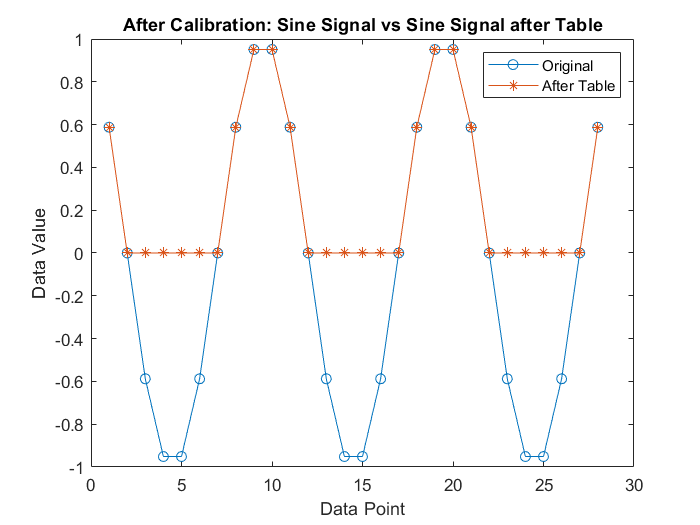Disconnect from the Server

To deactivate communication with the server, use the disconnect function. The XCP server can be safely closed after disconnecting.

disconnect(xcpCh)

clear a2lInfo

Vehicle Network Toolbox DocumentationGet trial now